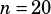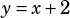Hi. Would it be possible for you to explain #13 on SAT practice test 5 on calculator inactive (the tea bag problem)? I think the main reason I found it confusing was the wording, and the SAT explanation for the answer was also a little bit wordy.
Thanks

I’ll try to be as un-wordy as possible! 🙂

I think plugging in numbers helps here. Say the restaurant wants to make 20 cups of tea one night, so. The equationtells us that they’d usetea bags to make 20 cups of tea.

Now, what if they wanted to make one more cup of tea (in our example, 21 cups instead of 20 cups)? Well, the equation tells us they’d use 23 tea bags to do that:So they needed 22 bags to make 20 cups, and 23 bags to make 21 cups. That’s one extra bag for one extra cup.

The other way to think about this is that they’re just giving you a linear equation and asking for the rate of change (i.e., the slope). Think of the equationas. That’s a line with a slope of 1 (and a y-intercept of 2, but we don’t need that for this question). A slope of 1 means for every change in x, you get an equal change in y. In other words, for every change in cups of tea, you get an equal change in tea bags.

Do either of those explanations help?